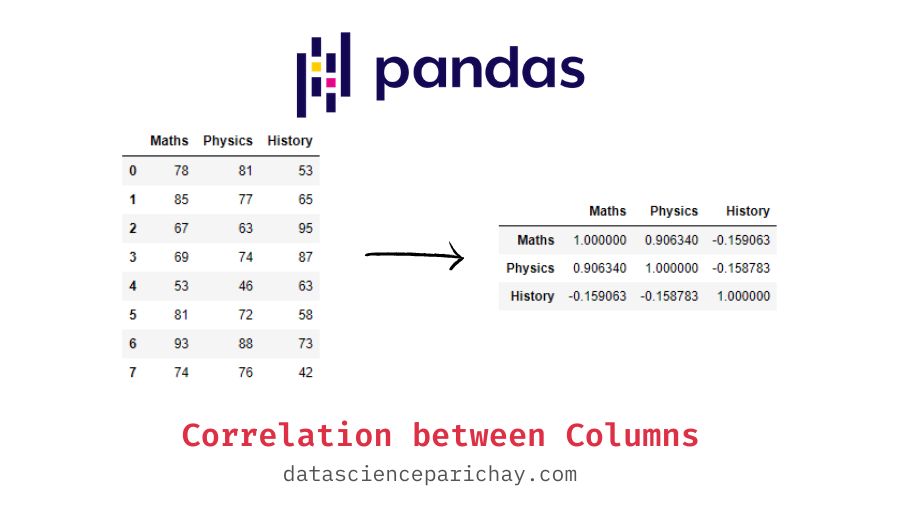# Get correlation between columns of Pandas DataFrame

Correlation is an important statistic that tells us how two sets of values are related to each other. A positive correlation indicates that the values tend to increase with one another and a negative correlation indicates that values in one set tend to decrease with an increase in the other set. In this tutorial, we will look at how to compute the correlation between two columns of a pandas dataframe.

You can use the pandas `corr()` function to get the correlation between columns of a dataframe. The following is the syntax:

```# correlation between Col1 and Col2
df['Col1'].corr(df['Col2'])```

If you are applying the `corr()` function to get the correlation between two pandas columns (that is, two pandas series), it returns a single value representing the Pearson’s correlation between the two columns. You can also apply the function directly on a dataframe which results in a matrix of pairwise correlations between different columns.

Let’s look at some examples to demonstrate the usage of the corr() function. First, we will create a sample dataframe that we will be using throughout this tutorial.

```import pandas as pd

# create dataframe
df = pd.DataFrame({
'Maths': [78, 85, 67, 69, 53, 81, 93, 74],
'Physics': [81, 77, 63, 74, 46, 72, 88, 76],
'History': [53, 65, 95, 87, 63, 58, 73, 42]
})

# display the dataframe
print(df)```

Output:

```   Maths  Physics  History
0     78       81       53
1     85       77       65
2     67       63       95
3     69       74       87
4     53       46       63
5     81       72       58
6     93       88       73
7     74       76       42```

We now have a dataframe storing the marks obtained by 8 high school students in subjects – Maths, Physics, and History. Let’s see if scores in one subject are correlated with scores in other subjects.

You can use the above syntax to directly get the correlation between two columns. For example, let’s see what is the correlation between the scores in Maths and Physics.

```# correlation between Maths and Physics
print(df['Maths'].corr(df['Physics']))```

Output:

📚 Data Science Programs By Skill Level

Introductory

Intermediate ⭐⭐⭐

🔎 Find Data Science Programs 👨‍💻 111,889 already enrolled

Disclaimer: Data Science Parichay is reader supported. When you purchase a course through a link on this site, we may earn a small commission at no additional cost to you. Earned commissions help support this website and its team of writers.

`0.9063395113712818`

We get ~0.91 as the correlation between the scores of Maths and Physics. This indicates that the two columns highly correlated in a positive direction. That is, for a higher value in Maths we are observing a higher value in Physics and vice versa.

You can also get the correlation between all the columns of a dataframe. For this, apply the `corr()` function on the entire dataframe which will result in a dataframe of pair-wise correlation values between all the columns.

```# pair-wise correlation between columns
print(df.corr())```

Output:

```            Maths   Physics   History
Maths    1.000000  0.906340 -0.159063
Physics  0.906340  1.000000 -0.158783
History -0.159063 -0.158783  1.000000```

When applied to an entire dataframe, the `corr()` function returns a dataframe of pair-wise correlation between the columns. We can see that there’s a weak negative correlation between scores of History and Maths/Physics. Also, notice that the values on the diagonal are 1s, this is because each column is perfectly correlated with itself.

Note that by default, the corr() function returns Pearson’s correlation. For more on the corr() function, refer to its documentation.

With this, we come to the end of this tutorial. The code examples and results presented in this tutorial have been implemented in a Jupyter Notebook with a python (version 3.8.3) kernel having pandas version 1.0.5

•